# Cyclic Symmetry

Cyclic symmetry allows you to analyze a model with circular patterns around an axis by modeling a representative segment. The segment can be a part or an assembly. The geometry, restraints, and loading conditions must be similar for all other segments (cyclically patterned) making up the model. Turbine, fans, flywheels, and motor rotors can usually be analyzed using cyclic symmetry.

In the Fixture PropertyManager under Advanced, select Cyclic symmetry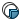.

The Cyclic symmetry restraint can be applied for static and nonlinear studies.

You define cyclic symmetry for two cut sections with the same area and shape. The number of segments that are repeated about the axis of revolution of the symmetry should be an integer number, which is the result of ( 360o / symmetry angle).

Selectable entities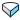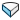Selection (face)

The faces on the cut section. The faces should be similar in shape and area and can be non-planar. The angle between the two faces should be evenly divisible by 360o.
More complex geometry may require a cut section with non-planar faces that intersect the axis. The section must be created in such a way that if the section is patterned using an equal spacing circular pattern, the resultant geometry forms a complete part without any gaps or interferences between the sections.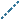Axis Axis of revolution for symmetry. The axis of symmetry must lie at the intersection of the two selected planes.

Nodes on opposite cut sections with similar relative positions displace similarly.

For example, locations with similar color of dots in the figure deform similarly. If tangential loads are applied, the cut sections with circular summery can deform normal to their planes.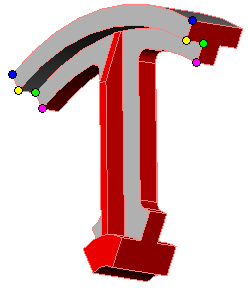Cyclic symmetry boundary conditions are constraint equations that are enforcing same displacements and rotations for the corresponding source and target nodes in a local cylindrical coordinate system defined by an axis of symmetry. In general Cyclic Symmetry constraint equations are adding stability to the body they are applied, but are not preventing the body from moving along or rotating about the axis of symmetry. It is recommended to apply the appropriate boundary conditions to stabilize the model and prevent rigid body modes when applying cyclic symmetry.

If tangential loads exist and the cut sections can deform normal to their planes, use Cyclic symmetry. If the model has a circular pattern and the loads are such that the cut sections cannot deform normal to their planes, you can analyze a representative pattern and apply Symmetry constraints on the cut sections.

## Example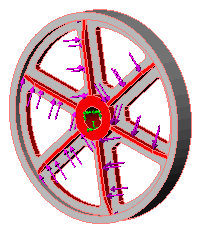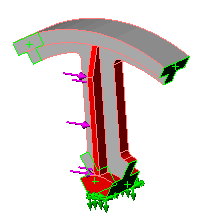There are six spokes in the part. A wedge angle of 60 degrees (360/6) centered on one of the spokes produces a section appropriate for cyclic symmetry. Apply cyclic symmetry to the cut faces.